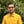Trusted answers to developer questions
Trusted Answers to Developer Questions

Related Tags

ruby
math
cos
communitycreator

# What is Math.cos() in Ruby?Harris Amjad

Grokking Modern System Design Interview for Engineers & Managers

Ace your System Design Interview and take your career to the next level. Learn to handle the design of applications like Netflix, Quora, Facebook, Uber, and many more in a 45-min interview. Learn the RESHADED framework for architecting web-scale applications by determining requirements, constraints, and assumptions before diving into a step-by-step design process.

The cos() function in Ruby calculates the cosine of a number. To be more specific, it returns the cosine of a number in radians.

Figure 1 shows the mathematical representation of the cos() function.

Figure 1: Mathematical representation of cosine function

Note:

• This function is present in the Math module.
• This cos() function only works for right-angled triangles.

## Syntax

cos(num)


## Parameter

This function requires a number that represents an angle in radians as a parameter.

To convert degrees to radians, use the following formula.

radians = degrees * ( pi / 180.0 )


## Return value

cos() returns the cosine of a number that is sent as a parameter. The return value is in the range [-1,1].

## Example

#positive number in radiansputs "The value of cos(2.3) : ",  Math.cos(2.3)#negative number in radiansputs "The value of cos(-2.3) : ",  Math.cos(-2.3)#converting the degrees angle into radians and then applying cos()#degrees = 180#PI = 3.14159265result = 180.0 * (Math::PI / 180.0)puts "The value of cos(#{result}) : ",  Math.cos(result)

RELATED TAGS

ruby
math
cos
communitycreator

CONTRIBUTORHarris Amjad

Grokking Modern System Design Interview for Engineers & Managers

Ace your System Design Interview and take your career to the next level. Learn to handle the design of applications like Netflix, Quora, Facebook, Uber, and many more in a 45-min interview. Learn the RESHADED framework for architecting web-scale applications by determining requirements, constraints, and assumptions before diving into a step-by-step design process.

Keep Exploring

Learn in-demand tech skills in half the time